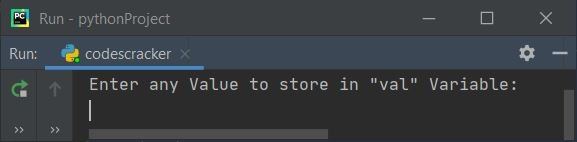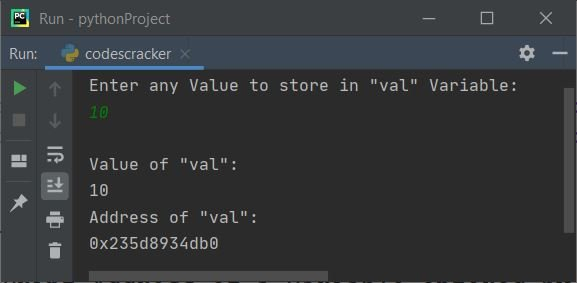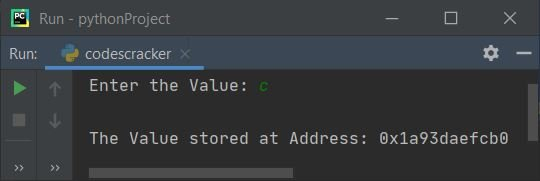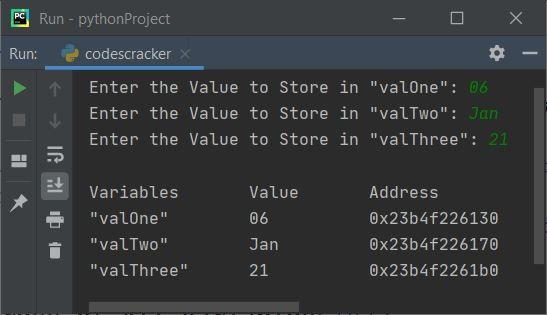# Python Program to Find and Print Address of Variable

• Print address of a variable
• Print address of multiple variables

## Find and Print Address of a Variable

The question is, write a Python program that find and prints address where the value entered by user stored in local computer system. Here is its answer. This program receives a number from user say 10 and the number gets initialized to a variable say val. Then the address of this variable val gets printed on output like shown in the program and its sample run given below:

```print("Enter any Value to store in \"val\" Variable: ")
val = input()
print("\nValue of \"val\":")
print(val)
print(hex(id(val)))```

Here is the initial output of this program's sample run:Now supply the input say 10 and press `ENTER` key to print the address of variable val where the given input is stored:#### Modified Version of Previous Program

The end used in this program, to skip insertion of an automatic newline using print().

```print(end="Enter the Value: ")
val = input()
print("\nThe Value stored at Address: " + hex(id(val)))```

Here is its sample run with user input, c:## Print Address of Multiple Variables

This program allows user to enter multiple values. And all these values gets initialized to its respective variables. Then finally the address of all these variables gets printed on output like shown in the program and its sample output given below:

```print(end="Enter the Value to Store in \"valOne\": ")
valOne = input()
print(end="Enter the Value to Store in \"valTwo\": ")
valTwo = input()
print(end="Enter the Value to Store in \"valThree\": ")
valThree = input()
print("\"valOne\"\t\t" +str(valOne)+ "\t\t\t" +str(hex(id(valOne))))
print("\"valTwo\"\t\t" +str(valTwo)+ "\t\t\t" +str(hex(id(valTwo))))
print("\"valThree\"\t\t" +str(valThree)+ "\t\t\t" +str(hex(id(valThree))))```

Here is its sample run with user input, 06, Jan and 21 as three inputs:This program uses list to receive any number of inputs and then prints all the input values with its address where those values gets stored.

```print(end="Enter the Size: ")
arrSize = int(input())
print(end="Enter " +str(arrSize)+ " Numbers: ")
arr = []
for i in range(arrSize):
arr.append(int(input()))

for i in range(arrSize):
print("arr["+str(i)+"]\t\t" +str(arr[i])+ "\t\t\t" +str(hex(id(arr[i]))))```

Here is its sample run with user input, 6 as size and 10, 20, 30, 40, 50, 60 as six numbers:Python Online Test

« Previous Program Next Program »